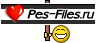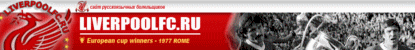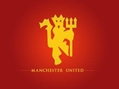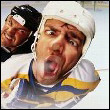Новые сообщения · Участники · Правила форума · Поиск · RSS
 Форум про Pes » Pro Evolution Soccer 2014 (PES 2014) » Все для Pes 2014 » ID игроков
ID игроков
TristeДата: Четверг, 2013-10-10, 13:36 | Сообщение # 1AdminГруппа: Администраторы
Сообщений: 1938
Награды: 48
Репутация: 12602
Статус: Offline

# ID игроков

Для вашего удобства выставляем ID игроков PES 2014. Скачать сможете по сылке ниже.

 DEANDREVIL Дата: Четверг, 2013-10-10, 13:43 | Сообщение # 2спасибоГруппа: Модераторы Сообщений: 122 Награды: 23 Репутация: 32767 Замечания: 0% Статус: Offline Ну вот же !!!, ДОЖДАЛИСЬ !!!, СПАСИБО !!!Triste Дата: Четверг, 2013-10-10, 13:44 | Сообщение # 3AdminГруппа: Администраторы Сообщений: 1938 Награды: 48 Репутация: 12602 Статус: Offline DEANDREVIL, Тебе спсОбложка:
 base55 Дата: Суббота, 2013-10-26, 19:56 | Сообщение # 4Группа: Пользователи Сообщений: 1 Награды: 0 Репутация: 0 Замечания: 0% Статус: Offline привет всем подскажите как поставить правильно татуировки???
 MAN_utd Дата: Воскресенье, 2013-11-17, 21:10 | Сообщение # 5Группа: Проверенные Сообщений: 14 Награды: 1 Репутация: 51 Замечания: 0% Статус: Offline спасибо тебе огромное Triste!!!
 Triste Дата: Воскресенье, 2013-11-17, 21:11 | Сообщение # 6AdminГруппа: Администраторы Сообщений: 1938 Награды: 48 Репутация: 12602 Статус: Offline MAN_utd,Обложка:
 Alex111 Дата: Пятница, 2014-01-03, 21:18 | Сообщение # 7Группа: Пользователи Сообщений: 5 Награды: 0 Репутация: 0 Замечания: 0% Статус: Offline подскажите кто нибудь после установки песедита 2.1 лица можно закидывать? если да то помогите... пож...
 Triste Дата: Пятница, 2014-01-03, 21:25 | Сообщение # 8AdminГруппа: Администраторы Сообщений: 1938 Награды: 48 Репутация: 12602 Статус: Offline Alex111, поищи ответ в комметах к патчу http://pes-files.ru/load....0-16354 Обложка:
 pesfan7 Дата: Суббота, 2014-06-14, 16:37 | Сообщение # 9Группа: Проверенные Сообщений: 2 Награды: 2 Репутация: 60 Замечания: 20% Статус: Offline ID игроков для патча PESEDIT [map] player=45893,"EL SHENAWY" player=57127,"Sayed Muawed" player=59955,"A. EID" player=32299,"EKRAMY" player=57123,"MOHAMED SALAH" player=57125,"shika" player=9487,"Hosni" player=59890,"Gomaa" player=47220,"ahmed hasan oka" player=47005,"A. HEGAZY" player=9515,"Artur Boruc" player=42552,"Nacer Chadli" player=40716,"Erik Lamela" player=40871,"Christian Eriksen" player=40937,"Wojciech Szczesny" player=6334,"Benaglio" player=41201,"Ralf" player=8823,"Guerrero" player=7312,"Daniel Alves" player=8820,"Raul Meireles" player=2138,"Pirlo" player=865,"Puyol" player=32836,"Agger" player=4152,"Rooney" player=4208,"Torres" player=8823,"Guerrero" player=32607,"Nani" player=3712,"Tevez" player=33702,"Aguero" player=55981,"Aguero" player=37306,"Balotelli" player=7959,"Fabregas" player=4072,"Valdes" player=9375,"Cazorla" player=40352,"Neymar" player=39173,"Thiago Silva" player=33799,"Hamsik" player=37106,"Di Maria" player=7814,"Dante" player=37448,"Buttner" player=36998,"Hazard" player=5726,"Hugo Almeida" player=7104,"Robinho" player=970,"Totti" player=37171,"Giovinco" player=9182,"Menez" player=5558,"Drogba" player=38568,"Busquets" player=33237,"Silva" player=37151,"Diamanti" player=4526,"Quaresma" player=7880,"Debuchy" player=38627,"Giroud" player=2586,"Ibrahimovic" player=32493,"Lavezzi" player=9133,"Pepe" player=4918,"Sneijder" player=34982,"Marchisio" player=15038,"Hulk" player=40350,"Boateng" player=36817,"Mandzukic" player=36867,"Coentrao" player=3603,"De Rossi" player=6526,"Podolski" player=8454,"Danny" player=32395,"Lichtsteiner" player=36356,"Kone" player=4522,"c.Ronaldo" player=40075,"Ramires" player=41126,"Thiago" player=9117,"Song" player=36718,"A.Sanchez" player=33702,"Aguero" player=5726,"Almeida" player=37306,"Balotelli" player=33079,"Bonucci" player=38568,"Busquets" player=9375,"Cazorla" player=36867,"Coentrao" player=7312,"DaniAlves" player=8454,"Danny" player=7814,"Dante" player=7880,"Debuchy" player=3522,"Defoe" player=3603,"DeRossi" player=37151,"Diamanti" player=45089,"Digne" player=37106,"DiMaria" player=5558,"Drogba" player=7959,"Fabregas" player=37171,"Giovinco" player=38627,"Giroud" player=4884,"GlenJohnson" player=33799,"Hamsik" player=2586,"Ibrahimovic" player=40350,"JBoateng" player=6483,"JJones"Добавлено (14.06.2014, 16:29)---------------------------------------------ПРОДОЛЖЕНИЕ ID игроков для патча PESEDIT player=36356,"Kone" player=40716,"Lamela" player=32493,"Lavezzi" player=7225,"Lescott" player=5848,"LuisFabiano" player=8878,"Maggio" player=36817,"Mandzukic" player=34908,"Marcelo" player=38055,"Marchetti" player=34982,"Marchisio" player=45993,"Marquinhos" player=8820,"Meireles" player=9182,"Menez" player=7511,"Messi" player=33225,"MSuarez" player=32607,"Nani" player=40352,"Neymar" player=41232,"Oscar" player=9133,"Pepe" player=6526,"Podolski" player=865,"Puyol" player=40075,"Ramires" player=7104,"Robinho" player=4152,"Rooney" player=33237,"Silva" player=4918,"Sneijder" player=3712,"Tevez" player=41126,"Thiago" player=4071,"Thiago Motta" player=39173,"ThiagoSilva" player=4208,"Torres" player=970,"Totti" player=4072,"Valdes" player=46003,"Verratti" player=36714,"Vidal" player=33700,"Walcott" player=38594,"Wilshere" player=45127,"Bertrand" player=32280,"Mikel" player=57472,"Willian" player=36877,"Diego Costa" player=59548,"Ayhan" player=45157,"Camapana" player=40887,"Djuricic" player=38852,"Faraoni" player=63506,"Holscher" player=43099,"R Miyaichi" player=44601,"Niang" player=42892,"S. Roberto" player=46842,"Weiser" player=39936,"Mkhitaryan" player=39744,"Lukasz Piszczek" player=45880,"Cristian Tello" player=38925,"Sandro" player=57123,"Mohamed Salah" player=43135,"Kovacic" player=35496,"Bale" player=42865,"Illarra" player=30113,"Palacio" player=40220,"Pastore" player=39704,"Armero" player=38051,"Jovetic" player=40428,"Andre Santos" player=59510,"Jese" player=36396,"Ivanovic" player=3176,"Schwarzer" player=42400,"Van Ginkel" player=9375,"Cazorla" player=7793,"Sanga" player=34005,"Negredo" player=30186,"Eduardo" player=38211,"Feghouli" player=43079,"Ghoulam" player=37144,"Matri" player=6772,"Diego Milito" player=63437,"Jodlowiec" player=319263,"Slimani" player=33643,"Benatia" player=37286,"Rodwell" player=34900,"Rafael Sobis" player=9308,"Diego Lopez" player=40639,"Muniain" player=40525,"Perin" player=45944,"Reus" player=34862,"Burak" player=5106, "Jefferson Farfan" player=8823, "Paolo Guerrero" player=38796,"Alderweireld" player=42556,"Isco" player=38082,"Ramsey" player=36888,"Caceres Martin" player=37536,"Asamoah Kwadwo" player=32828,"Skrtel" player=4865,"Kolo Toure" player=42664,"Mignolet" player=38945,"Ba" player=33926,"Guarin" player=36990,"Brahimi" player=42669,"Casemiro" player=59504,"Zuber"Добавлено (14.06.2014, 16:30)---------------------------------------------ПРОДОЛЖЕНИЕ ID игроков для патча PESEDIT player=42898,"Lucas Moura" player=268479124,"Oscar Romero" player=57005,"Sercan Sararer" player=32354,"KPBoateng" player=42675,"Paulinho" player=5430,"Lennon" player=40753,"JacksonMar" player=40749,"Teo" player=60263,"Eder Balanta" player=44857,"Santiago Arias" player=31044,"Falcao" player=40631,"Cuadrado" player=36704,"Zuniga" player=38907,"S.Ibarbo" player=43170,"Townsend" player=40347,"Khedira" player=45690,"J Quintero" player=1498,"Eto'O" player=1073758633,"Akino" player=41021,"Aurtenetxe" player=44383,"Courtois" player=43288,"Savic" player=1697,"Santa Cruz" player=34098,"Modric" player=46666,"Iago Aspas" player=36862,"Oscar Cardozo" player=7511,"Lionel Messi" player=4039,"Fabio" player=268444600,"Fred" player=47727,"Alex Telles" player=43075,"Kondogbia" player=45011,"Michu" player=4522,"C.Ronaldo" player=32199,"Quagliarella" player=34974,"Ogbonna" player=42574,"Y.Sanogo" player=42552,"N.Chadli" player=44925,"Yero" player=4072,"Valdes" player=38845,"Benteke" player=47070,"Shaw" player=42641,"coutinho" player=41046,"Nainggolan" player=33633,"Higuain" player=866,"Xavi" player=1653,"Pizarro" player=293204,"Fedetskyi" player=38261,"Yarmolenko" player=38655,"Canales" Player=58843,"Moreno" Player=116238,"Ake" player=2586,"Ibrahimovic" player=30122, "Gago" player=40663, "Erbes" player=41522, "Burrito Martinez" player=42694, "L. Marin" player=45254, "Sanchez Mino" player=45356, "L. Paredes" player=60137, "Gigliotti" player=35207, "David Luiz" player=7444, "David Villa" player=9129,"Ribery" player=31044,"Falcao" player=38567,"Pedro" player=8978,"Handanovic" player=42429,"Juan Jesus" player=35476,"Kuzmanovic" player=41537,"Ricky Alvarez" player=38568,"Sergio Busquets" player=38699,"A. Pereira" player=44702,"Barkley" player=57353,"Carvajal" player=63457,"Luis Alberto" player=32339,"Nuri Sahin" player=46637,"Mauro Icardi" player=47006,"I.Mbaye" player=57427,"Wallace" player=5038,"Mudingay" player=7063,"Carizzo" player=32062,"Andreolli" player=40228,"Obi" player=268481212,"Gustavo Gomez" player=305518,"Konoplyanka" player=43040,"Gotze" player=32313,"Dembele" player=37017,"Sakho" player=5627,"De Jong" player=45880,"Cristian Tello" player=7959,"Fabregas" player=7103,"Alex" player=32493,"Lavezzi" player=63398,"Morrison" player=46554,"Chiriches" player=40122,"Lukaku" player=45685,"J Quintero" player=44636,"Muriel" player=41640,"Carlos Bacca" player=5050,"Perea" player=33114,"Cristian Zapata" player=39478,"Ranocchia" player=43094,"Morata" player=9317,"Arda Turan" player=57438,"Hernane" player=46303,"Gabriel" player=3655,"Leo Moura" player=4769,"Luisao" player=35446,"Maxi" player=40240,"Matic" player=36862,"Oscar Cardozo" player=4073,"Iniesta" player=36764,"Kolarov" player=37638,"Dzeko" player=44776,"Nastasic" player=9611,"Juan Vargas" player=35477,"Cavani" player=31221,"Valencia" player=34881,"Luis Suarez" player=38499,"Strootman" player=5648,"Hamit" player=44709,"Berahino" player=41425,"Eduardo Vargas" player=46181,"Jiracek" player=38477,"Kozak" player=45011,"Michu" player=990,"Nedved" player=47431,”Dier” player=57413,”Vydra” player=4771,"Howard" player=46235,"L. Insigne" player=40254,"Ljajic" player=4398,"De Sanctis" player=40254,"Ljajic" player=4739,"Ignashevich" player=268476117,"Lucas Pratto" player=319263,"Slimani" player=304746,"Stepanenko" player=302987,"Denis Garmash" player=,"Edmar" player=60009,"Gonzalez Ferreira" player=59766,"Ake" player=39325,"Wilfried" player=34007,"Valero" player=47785,"Stones" player=43445,"Lars Bender" player=43133,"Luiz Gustavo" player=43449,"Sidney Sam" player=15408,"Doumbia" player=40709,"Belfodil" player=9341,"Mario Gomez" player=35014,"Constant" player=268483465,"Angel Romero" player=8843,"Pitroipa" player=42868,"Bartra" player=35539,"Bougherra" player=38081,"Kieran Gibbs" player=32441,"Anderson" player=31052,"Fernandinho" player=42874,"Nacho Fernandez" player=5800,"Chamakh" player=37159,"Poli" player=35878,"Lucas Leiva" player=35173,"Andy Carroll" player=5440,"Borriello" player=43751,"Marquinho" player=2315,"Burdisso" player=37250,"Leandro Castan" player=268477580,"Pittoni" player=43669,"Rojas" player=40532,"H.Perez" player=268475166,"E.Benitez" player=268479122,"D.Ortiz" player=33699,"G.Dos Santos" player=37726,"Mphela" player=1737,”J.Zanetti” player=30113,”Palacio” player=6265,"Afellay" player=41451,"Charles Aranguiz" player=42751, "Eugenio Mena" player=40507,”Lacazette” player=38190,”Boudebouz” player=34993,”Bisevac” player=46481,”Khazri” player=41000,”Cabella” player=38022,”Cvitanich” player=33813,”Erding” player=9418,”Gourcuff” player=4007,”Aliadiere” player=57207,”Kurzawa” player=46805,"Kebba Ceesay" player=46741,"Gergo Lovrencsics" player=63218,"Osmar Molinas" player=268475162,"F.Montero" player=42538,"Rodrigo" player=46834,"Deulofeu" player=46637,"Icardi" player=5069,"Pinilla" player=41479,"Felipe Gutierrez" player=3758,"Kaka" player=30242,"Gelso Ortiz" player=40925,"Alex Teixeira"Добавлено (14.06.2014, 16:32)---------------------------------------------ПРОДОЛЖЕНИЕ ID игроков для патча PESEDIT player=46487,"Thauvin" player=35017,"Gignac" player=42246,"Aboubakar" player=42336,"Hamouma" player=34991,"Monnet paquet" player=7866,"Obraniak" player=47786,"Lemina" player=34102,"Ochoa" player=37021,"Sankhare" player=35031,"Mongogu" player=34004,"Barral" player=41082,"Koke" player=2052,"Distin" player=47406,"Ruben Garcia" player=40229,"Destro" player=32190,"Balzaretti" player=41200,"Dodo" player=60627,"William Carvalho" player=44852"Kelvin" player=41174"Rojo" player=45139,"Taider" player=37291,"Begovic" player=54683,"Bicakcic" player=37638,"Dzeko" player=30233,"Salihovic" player=33374,"Spahic" player=58640,"Zukanovic" player=43467, "Rodriguez" player=8940,"BenArfa" player=46466,"Mandi" player=38190,"Boudebouz" player=60833,"Jean Deza" player=34007,"Borja Valero" player=6840,"Marica" player=57025,"Maxim" player=35548,"Radu" player=1287,"Mutu" player=300405,"Yarmolenko" player=322087,"Zozulya" player=42602,"Stepanenko" player=40850,"Douglas Costa" player=30186,"Eduardo" player=42892,"S.Roberto" player=7959,"Fabregas" player=38567,"Pedro" player=38568,"Sergio Busquets" player=8639,"Pique" player=38013,"Gebre" player=38740,"Grenier" player=41082,"Koke" player=40507,"Lacazette" player=40002,"Lewandowski" player=44601,"Niang" player=43063,"SonHeungMin" player=38594,"Wilshere" player=46235, "L.Insigne" player=42869, "Montoya" player=40632,"Belhanda" player=40602,"Khacheridi" player=34997,"Tremoulinas" player=40843,"Harmash" player=5742,"Gusiev" player=40985,"Ideye" player=31019,"MIGUEL VELOSO" player=42592,"Koval" player=43540,"Vida" player=36374,"Vukojevic" player=7329,"Ramos" player=54444,"Aubameyang" player=46532,"Lambert" player=41006,"BOYATA" player=37918,"Guzan" player=47076,"Cornelius" player=8846,"Dejagah" player=35019,"Remy" player=37433,"Fer" player=47238,"Clyne" player=9193,"Filipe" player=34475,"Godin" player=40240,"Matic" player=32586,"Miranda" player=36867,"Coentrao" player=4208,"Torres" player=37000,"Adil Rami" player=38796,"Alderweireld" player=43041,"Holtby" player=40122,"Lukaku" player=42552,"N.Chadli" player=3577,"Heitinga" player=40065,"Mertens" player=43467,"Rodriguez" player=54715,"Lasogga" player=43445,"Lars Bender" player=43449,"Sidney Sam" player=53916,"Volland" player=54659,"Aigner" player=54337,"Arnold" player=54664,"Joselu" player=54116,"Kruse" player=54431,"Sven Bender" player=37155,"Campagnaro" player=38439,"Perisic" player=44383,"Courtois" player=60884,"Gnabry" player=33633,"Higuain" player=57123,"Salah" player=36358,"Sokratis" player=2530,"Van Buyten" player=39327,"VKadlec" player=38297,"Willian" player=40220,"Pastore" player=4261,"Mexes" player=33861,"Nocerino" player=34892,"Birsa" player=6653,"Montolivo" player=35880,"E.Sosa" player=42346,"Silvio" player=7444,"Villa" player=7303,"Gabi" player=33225,"M.suares" player=35097,"Adrian" player=47051,"Rabiot" player=63520,"Cristante" player=4414,"Muntari" player=822,"Gerrard" player=47711,"Martial" player=57350,"Moises Gomez" player=45725,"Aquino" player=40753,"JacksonMar" player=40631,"Cuadrado" player=35457,"Macnelly Torres" player=40631,"Cuadrado" player=37039,"Carlos Sanchez" player=54590,"Adrian Ramos" player=30324,"Congre" player=44661,"Rodelin" player=43985, "Alex Sandro" player=42538, "Rodrigo" player=42402,"Josue" player=4526 Quaresma" player=42349,"Lima" player=42348,"Pizzi" player=44852,"Kelvin" player=59590,"bakkali" player=47390,"Mendes" player=36877,"Diego Costa" player=8944,"Benzema" player=36797,"Mitroglou" player=8474,"Barnetta" player=41083,"Salvio" player=41083,"Salvio" player=45076,"Zouma" player=44455,"Corgnet" player=45076,"Diabate" player=38194,"Tabanou" player=15893,"Kiyotake" player=43202,"Neto" player=33700,"Walcott" player=42675,"Paulinho" player=44776 ,"Nastasic" player=47276,"Bosetti" player=33926,"Guarin" player=55444,"Aubameyang" player=9000, "Pelle" player=47443, "Boetius" player=38032, "Immers" player=38036, "Janmaat" player=37430,"Siem" player=37086,"Kelly" player=41004,"Borini" player=60002,"R.Saponara" player=45404,"Ferri" player=36766,"Mata" player=63309,"Zaza" player=70505,"Sanabria" player=36766,"Mata" player=9319,"Ziegler" player=43206,"Kurtic" player=59732,"Lodygin" player=36766,"Mata" player=63730,"Diego" player=41609,"Abdennour" player=45267,"Suso" player=45051,"sansone" player=54342,"Dost" player=55540,"Devic" player=46987,"Benzia" player=42554,"Bengtsson" player=35321,"P.Bengtsson" player=8899,"Perez" player=268470722,"Pato" player=42378,"Dusan Tadic" player=63424,"Lins" player=42455,"Ganso" player=54341,"Diego Ribas" player=43094,"Morata" player=37144,"Matri" player=41066,"Immobile" player=42653,"Cholebas" player=38845,"Benteke"Добавлено (14.06.2014, 16:33)---------------------------------------------ПРОДОЛЖЕНИЕ ID игроков для патча PESEDIT player=32159,"Matuidi" player=38240,"Ozil" player=30447,"Cabaye" player=33794,"Osvaldo" player=38627,"Giroud" player=32354,"KPBoateng" player=40875, "De Vrij" player=42375, "Indi" player=42440, "Clasie" player=46859, "Vilhena" player=41124,"Alaba" player=46531,"Lallana" player=43041,"Holtby" player=41000 ,"Cabella" player=36797,"Mitroglou" player=42574,"Y.Sanogo" player=43170 ,"Townsend" player=4650 ,"Kallstrom" player=30277,"Llorente" player=820,"Ferdinand" player=7836,"Flamini" player=33861,"Nocerino" player=35477,"Cavani" player=36770,"Kroos" player=36797,"Mitroglou" player=37416,"Capoue" player=42898,"Moura" player=33870,"Bendtner" player=59481,"Berardi" player=38120,"Callejon" player=46564,"Darida" player=47306,"Finnbogason" player=42101,"Iturbe" player=45023,"Pogba" player=52947,"Blanco" player=59733,"Volovyk" player=42838,"Andre" player=36773,"Bolatti" player=*****,"Moledo" player=46037,"Bernard" player=43786,"Fernando" player=36320,"Seleznyov player=34813,"Arnautovic" player=34614,"Sidwell" player=9044,"Jones" player=37294,"Tomkins" player=54115,"L.de Jong" player=40278,"NZonzi" player=44355,"Snodgrass" player=39273,"Yanga Mbiwa" player=33167,"De Guzman" player=3682,"Demichelis" player=57553,"Dudu" player=35532,"Defour" player=35189,"Taarabt" player=38346,"Dejan Lovren" player=38345,"Badelj" player=59546,"Kirchhoff" player=268481426,"Ademilson player=268472277,"Barcos" player=5134,"Eguren" player=268465885,"Jadson" player=43297,"Jefferson" player=268481490,"Neto Berola" player=46194,"Ygor" player=36945,"Fagner" player=35335,"Blaszczykowski" player=46825,"Emre Can" player=60622,"Braithwaite" player=54670,"Oczipka" player=38843,"Mangala" player=57634,"Movsisyan player=38947,"Dzagoev" player=42683,"Wallyson" player=59547,"Holbjerg" player=44685,"Mustafi" player=54665,"Jung" player=46536,"Ward-Prowse" player=70482,"Nathan Ake" player=43154,"Nathan Redmond" player=45135,"Sammy Ameobi" player=38489,"Assaidi" player=42408,"Kasami" player=43254,"Puncheon" player=44682,"Wanyama" player=32578,"Mirallas" player=37469,"Iakovenko" player=34698,"Bastos" player=39729,"Subotic" player=37960,"Faizullin" player=268437087,"Ronaldinho" player=32454,"Romaric" player=6337,"Lahm" player=9571,"Alberto Rodriguez" pplayer=60534,"Januzaj" player=37827,"Witsel" player=48103,Reimond Manco player=36556,Chicharito player=43187,"Ola John" player=9133,"Pepe" player=35108,"JaviMartinez" player=54672,"Rode" player=54429,"Weidenfeller" player=1653,"Pizarro" player=33643,"Benatia" player=45290,"Inigo" player=35553,"Hernanes" player=42429 ,"Juan Jesus" player=36770,"Kroos" player=54430,"Grosskreutz" player=301873,"Subotic" player=5106,"Farfan" player=40122,"Lukaku" player=8946,"Nasri" player=60485,"Rekik" player=34982,"Marchisio" player=30277,"Llorente" player=8822,"Rossi" player=8957,"Cerci" player=38499,"Strootman" player=41153,"Coates" player=36703,"Ospina" player=38907,"Ibarbo" player=32092,"Soto" player=47965,"Pedro Franco" player=60424,"Johan Mojica" player=60513,"Humberto Osorio" player=59879,"Mondragon" player=33266,"C.Davies" player=8846,"DEJAGAH" player=43041,"HOLTBY" player=33198,"DIAME" player=34848,"S. MARVEAUX" player=37201,"ROSE" player=34841,"Sessegnon" player=33287,"Tiote" player=59779,"E.Ba" player=37201,"ROSE" player=8760,"Routledge" player=31483,"Ron Vlaar" player=61175,"T.Ince" player=54716,"Mancienne" player=63607,"Fabinho" player=46828,"Alcacer" player=37470,"Gillet" player=44659,"Krychowiak" player=30210,"Yaya Toure" player=44834,"van rhijn" player=54757,"Leitner" player=54590,"Adrian Ramos" Player=40749,"Gutierrez" Player=43379,"C. Carbonero" Player=60263,"Eder Balanta" Player=40631,"Maidana" Player=41518,"M. Barovero" player=47096,"Weihrauch" player=43042,"Schuerrle" player=4934,"Schweinsteiger" player=39216,"Moses" player=37106,"DiMaria" player=46840,"Powell" player=7106,"Leo" player=3712,"TEVEZ" player=41125,"Gedo" player=41609,"ABDENNOUR" player=319187,"Ahmed Yaseen Gheni" player=60553,"Samuel Garcia" player=319298,"Yousif MSEKNI" player=319299,"Walid HICHERI" player=3758,"Kaka" player=34982,"Marchisio" player=30277,"Llorente" player=8822,"Rossi" player=8957,"Cerci" player=38499,"Strootman" player=268483081,"Alejandro Silva" player=42931,"Musa" player=40340,"Koscielny" player=15433,"Yoshida" player=15408,"Doumbia" player=11055,"Honda" player=6408,"Mertesacker" player=43148,"Lindegaard" player=36998,"Hazard" player=8957,"Cerci" player=4013,"Evra" player=39755,"A.Williams" player=7817,"Mathieu" player=37336,"Candreva" player=37430,"Siem" player=37086,"Kelly" player=41004,"Borini" player=42550,"Sigthorsson" player=46598,"Butland" player=8921,"Ruben Castro" player=45076,"Zouma" player=47163,"Victor Fischer" player=30158,"M.Kadlec" player=54433,"Hofmann" player=70417,"Freidirich" player=7030,"Silvestre" player=33734,"Aduriz" player=47248,"Baptistao" player=60534,"Januzaj" player=60491,"Fausto Rossi" player=42424,"Xhaka" player=,"Polomat" player=57676,"Gokhan Tore" player=3598,"Taddei" player=40871,"Eriksen" player=7507,"Juanfran" player=34475,"Godin" player=35109,"Benat" player=45725,"J.Aquino" player=61181,"Jesse Lingard" player=46834,"Deulofeu" player=46456,"Ghilas" player=3848,"Enyeama" player=57144,"Obaoabona" player=44345,"Ambrose" player=32280,"Mikel" player=3841,"Ejide" player=57141,"Agbim" player=45391,"Omeruo" player=57143,"Egwuekwe" player=3848,"Enyeama" player=45985,"Emenike" player=7866,"Obraniak" player=43443,"Reinartz" player=45144,"Leno" player=54711,"Jansen" player=42316,"Griezmann" player=37094,"Sturridge" player=36766,"Mata" player=58691,"Gabriel Paulista" player=40569,"Mccarthy" player=9185,"Sow" player=59924,"Sapara" player=57591,"Erten" player=3764,"Isaksson" player=5786,"Arshavin" player=46546,"Milanov" player=5024,"Kuyt" player=70463,"Bentaleb" player=35092,"Bruno" player=47335,"Eysseric" player=70383,"Rochina" player=57205,"Germain" player=34787,"Obbadi" player=33184,"Rafinha" player=32046,"Raggi" player=9138,"Moutinho" player=7812,"Toulalan" player=44756,"Gabbiadini" player=45950,"Wolski" player=40665,"J.Dos Santos" player=30345,"Dawson" player=2089,"Van Der Vaart" player=54254,"Karius" player=57265,"Giannelli Imbula" player=38943,"Fofana" player=34537,"Joris Marveaux" player=45112,"Jebbour" player=33808,"Campbell" player=47383,"Bowery" player=42376,"Kieftenbeld" player=46532,"Lambert" player=44820,"de Leeuw" player=42535,"Cedric" player=5537,"Barzagli" player=40347,"Khedira" player=7812,"Toulalan" player=45004,"Fabiano" player=44466,"Paulo Lopes" player=41163,"Enzo Perez" player=6314, "Demouge" player=43042,"Schuerrle" player=44562,"N Dyer" player=38635,"Cillessen" player=54437,"Kehl" player=7834,"Adebayor" player=34126,"Paletta" player=43680 ,"D.Reyes" player=30189,"Behrami" player=40525,"Perin" player=47070,"Shaw" player=9242 ,"R.Garcia" player=46536,"Ward-Prowse" player=268482024,"Erazo" player=41517,"Mugni" player=58665,"Leom" player=35496,"Bale" player=40753,"J.Martinez" player=57633,"Ozbiliz" player=*****,"Voronin" player=39550, "Kokorin" player=37432, "Dzsudzsak" player=39541, "Granat" player=35226, "Gabulov" player=39473, "Mehmedi" player=297022,"Lens" player=32618,"Schone" player=44407,"J. Campbell"Добавлено (14.06.2014, 16:35)---------------------------------------------ПРОДОЛЖЕНИЕ ID игроков для патча PESEDIT player=57288,"Bance" player=8984,"Gyan" player=59995,"Herrera" player=43680 ,"D.Reyes" player=45004 ,"Fabiano" player=40725 ,"Otamendi" player=43985 ,"AlexSandro" player=46418 ,"Lica" player=40407 ,"Maicon M" player=45142,"Kacaniklic" player=8374 ,"I. Uche" player=58843 ,"Alberto Moreno" player=58789,"Paulinho.n" player=41517,Lucas Mugni" player=58663,"Everton" player=58786,"Samir" player=57445,"Nixon" player=35487,"Biabiany" player=41174,"Rojo" player=57700,"Alan" player=38797,"Blind" player=45393,"Klaassen" player=39339,"Klaus" player=268475260,"M.Silva" player=36844,"Derdiyok" player=46850,"Veltman" player=70967,"Pektemek" player=35500,"Kavlak" player=822,"Gerrard" player=37094,"Sturridge" player=59949,Zambrano player=44653,"Mostefa" player=852,"Casillas" player=884,"Anelka" player=4685,"Hangeland" player=32839,"Grindheim" player=36666,"Criscito" player=39544,"D.Kombarov" player=2717,"Donovan" player=39201,"R.Elm" player=57685,"Kevin Kampl" player=57628,"K.Kombarov" player=46531,"Lallana" player=38765,"Lustig" player=43784,"Mario Fernandes" player=37830,"I. Popov" player=1066,"Riise" player=39719,"S. Rondon" player=6080,"Schaars" player=39414,"Shchennikov" player=41063,"Seferovic" player=2089,"Van Der Vaart" player=44834,"van Rhijn" player=31483,"Vlaar" player=35397,"Wernbloom" player=58640,"Zukanovic" player=57347,"Perbet" player=54727,"Zieler" player=40607,"Bardi" player=45190,"Livaja" player=37907,"Bryan Ruiz" player=46879,"Depay" player=40742,"Dragovic" player=61048,"Carlos Mane" player=44181,"Jardel" player=46843,"Neustaedter" player=44337,"Ibrahima Traore" player=42245,"Choupo-Moting" player=54662,"Constant Djakpa" player=54591,"Sami Allagui" player=59760,"Zaha" player=38491,"L.De Jong" player=42641,"Coutinho" player=42375,"Martins Indi" player=9591,"Aogo" player=46648,"Fahrmann" player=47162,"Kolasinac" player=45946,"Draxler" player=63408,"Felipe Santana" player=46651,"Hoger" player=37535,"Annan" player=60380,"Goretzka" player=41134,"Obasi" player=39976,"Szalai" player=47695,"Meyer" player=8421,"Huntelaar" player=63099,"Hoogland" player=59549,"Clemens" player=40393,"Duarte" player=852,"Casillas" player=43076,"Varane" player=40065,"Mertens" player=15430,"Araujo" player=53787,"Ginter" player=46850,"Veltman" player=34432,"Dzemaili" player=44678 ,"Wickham" player=38366,"Bonaventura" player=54435,"Durm" player=34783,"Lucas Biglia" player=42316,"Griezmann" player=4251,"Alonso" player=42892,"S. Roberto" player=32478,"Mbolhi" player=40295,"J.Henderson" player=46825,"Emre Can" player=44720,"Flanagan" player=3604,"Aquilani" player=36888,"Caseres M" player=40631,"Cuadrado" player=34939,"Diakite" player=54410,"Elia" player=42888,"Ilicic" player=40989,"Aurier" player=46536,"Ward-Prowse" player=63489,"Promes" player=39777,"K.Navas" player=37401,"Elderson" Player=45391,"Omeruo" player=40717,"Felipe Menezes" player=41076,"Josimar" player=45970,"Ademilson" player=57438,"Hernane" player=41231,"Jean" player=42683,"Wallyson" player=45970,"Ademilson" player=*****, "Minala" player=43277,"Lulic" player=6732,"Ledesma" player=34962,"Konko" player=47203,"Onazi" player=60563,"Balde Keita" player=37507,"Gonzalez" player=42816,"Cavanda" player=47007,"Duncan" player=47279,"Benassi" player=40709,"Belfodil" player=47006,"I.Mbaye" player=42063,"Mesbah" player=4039,"Fabio" player=59501,"Izet Hajrovic" player=60987,"Salih Dursun" player=60986,"Umut Gundogan" player=70429,"Bamford" player=42660,"van Aanholt" player=35123,"Fazio" player=8076,"Vanden Borre" player=32498,"Lacen" player=38671,"Djebbour" player=47085,"Tatos" player=35495,"Inler" player=6990,"Gokhan Zan" player=7084,"Melo" player=30209,"Eboue" player=36996,"Chedjou" player=37499,"Hakan Balta" player=39639,"Selcuk Inan" player=39640,"Umut Bulut" player=43070,"Yekta Kurtulus" player=5029,"Vidic" player=42352,"Phill Jones" player=15320,"Kagawa" player=61181,"Jesse Lingard" player=4013,"Evra" player=,"J.Wilson" player=8949,"Juan Arango" player=61068,"Sinkgraven" player=47002,"Ziyech" player=30173,"Diego Lugano" player=57549,"Bogdanov" player=57575,"Krasnopyorov" player=57572,"Goryainov" player=57508,"Giuliano" player=57504,"Boyko" player=57509,"Kankava" player=47594,"Ruben Botta" player=57587,"Gomenyuk" player=57611,"Ksenz" player=57577,"Marlos" player=57584,"Villagra" player=57570,"Devic" player=57579,"Moledo" player=54706,"Hakan Calhanoglu" player=54369,"Halil Altintop" player=54109,"Herrmann" player=54677,"Stendera" player=39619,"Edmar" player=57804,"Michy Batshuayi" player=45299,"De Sciglio" player=6566,"Abate" player=44868,"van Aanholt" player=35123,"Fazio" player=8076,"Vanden Borre" player=44608,"Wakaso" player=46031,"Leando Donizete" player=46027,"Pierre" player=70470,"Mahrez" player=60443,"Mendy" player=4178,"Malbranque" player=57629,"jurado" player=57813,"Ezekiel" player=36403,"Ryzhikov" player=41111,"Cork" player=38082,"Ramsey" player=40571,"De Gea" player=54123,"Ter Stegen" player=54727,"Zieler" player=38797,"Blind" player=33799,"Hamsik" player=40240 ,"Matic" player=4494,"Tiago" player=46834,"Deulofeu" player=41106,"Oviedo" player=36625 ,"Rakitic" player=43079,"Ghoulam" player=34881,"Suarez" player=37638,"Dzeko" player=42865,"Illarra" player=41311,"Basanta" player=37304,"Stuani" player=59927,"Caicedo" player=43274,"Mujdza" player=47698,"Alen Halilovic" player=47244,"Rafinha Alcantara" player=42852,"Moscardelli" player=60391,”Rafa” player=4769,”Luisao” player=33185,”Neuer” player=57027,”Markovic” player=36727,”Muslera” player=40295,”Henderson” player=5850,"Marcos Gonzalez" player=41428,"Marcelo Diaz" player=39701,"Jean Beausejour" player=39699,"Gary Medel" player=34489,"Gonzalo Jara" player=9612,"Jorge Valdivia" player=9567,"Claudio Bravo" player=45668,"Junior Fernandes" player=36714,"Arturo Vidal" player=42751,"Eugenio Mena" player=32780,"Pepe Rojas" player=37337,"Mauricio Isla" player=3726,"Johnny Herrera" player=41425,"Eduardo Vargas" player=5069,"Mauricio Pinilla" player=41451,"Charles Aranguiz" player=41468,"Gato Silva" player=41449,"Miiko Albornoz" player=57620,"Salvatore Bocchetti player=57433,"Fred De Paula" player=41589,"Facundo Ferreyra" player=2506,"Reina" player=43611,"Bedoya" player=47676,"Aron Johannsson" player=53898,"Chandler" player=61045,"Ivan Cavaleiro" player=60391,"Rafa Silva" player=57027,"L.Markovic" player=40295,"J. Henderson" playre=39377,"Glushakov" playre=37535,"Annan" playre=63408,"Felipe Santana" player=1001,"Rosicky" player=36288,"Wijnaldum" player=57158,"Ben Youssef" player=8421,"Huntelaar" player=1073787750,"Msakni" player=9421,"Bedimo" player=8552,"Kiessling" player=57304,"S. Mane" player=54447,"Verhaegh" player=41112,"Guedioura" player=40500,"Kadir" player=5420,"Medjani" player=61417,"Djabou" player=70500,"Jojic" player=4934,"Schweinsteiger" player=39215,"Renato Augusto" player=9144,"Fred" player=8823,"Guerrero" player=35266,"Pato" player=42675,"Paulinho" player=450,"Emerson" player=40659,"Kardec" player=61093,"Bazelyuk" player=60446,"Coman" player=54413,"Aaron Hunt" player=59509,"Vitolo" player=39259,"Jay Rodriguez" player=39193,"Subasic" player=42898,"Lucas Moura" player=3712,"Tevez" player=35207,"David Luiz"Добавлено (14.06.2014, 16:37)---------------------------------------------ПРОДОЛЖЕНИЕ ID игроков для патча PESEDIT player=5402,"Hoarau" player=39271,"Jourdren" player=8871,"Montano" player=39276,"El Kaoutari" player=46953,"Alessandrini" player=45467,"Lautoa" player=32038,"Brison" player=44072,"Sissoko" player=38181,"Nkoulou" player=46370,"Lucas Mendes" player=44665,"Khlifa" player=8707,"Gomis" player=33029,"Romao" player=57265,"Imbula" player=8559,"Audard" player=34605,"Traore" player=42576,"Ecuele Manga" player=35013,"Sall" player=43080,"Saadi" player=35002,"Ruffier" player=9115,"Cohade" player=60550,"Saint Maximin" player=30324,"Congre" player=7642,"Hilton" player=45255,"Ocampos" player=39226,"Corchia" player=44434,"Dja djedje" player=37021,"Sankhare" player=35031,"Mongogu" player=47786,"Lemina" player=34102,"Ochoa" player=42336,"Hamouma" player=34991,"Monnet paquet" player=7866,"Obraniak" player=42246,"Aboubakar" player=35017,"Gignac" player=34993,"Bisevac" player=38190,"Boudebouz" player=46481,"Khazri" player=38022,"Cvitanich" player=33813,"Erding" player=4007,"Aliadiere" player=57207,"Kurzawa" player=46487,"Thauvin" player=39262,"Roux" player=57213,"Carrasco" player=37219,"Djordjevic" player=60445,"Ongenda" player=38941,"Miguel Lopes" player=38442,"Riviere" player=2951,"S. Camara " player=35010,"Apam" player=9418,"Gourcuff " player=3848,"Enyeama" player=45320,"Gradel" player=41000,"Cabella" player=57616,"Dykan" player=57625,"Carioca" player=57627,"Tino Costa" player=57633,"Ozbiliz" player=57626,"Romulo" player=40348,"Ebert" player=57631,"Yakovlev" player=57632,"Kozlov" player=57634,"Movsisyan" player=57621,"Tasci" player=57623,"Parshivlyuk" player=57622,"Joao Carlos" player=57624,"Makeev" player=57617,"Rebrov" player=37028,"AAyew" player=2150,"Abbiati" player=7834,"Adebayor" player=30238,"Adriano" player=32836,"Agger" player=33702,"Aguero" player=38796,"Alderweireld" player=5726,"Almeida" player=5657,"Amauri" player=37306,"Balotelli" player=35250,"Banega" player=44702,"Barkley" player=804,"Bellamy" player=33870,"Bendtner" player=31979,"Bent" player=1310,"Berbatov" player=45127,"Bertrand" player=33079,"Bonucci" player=38568,"Busquets" player=37448,"Buttner" player=40724,"CarlosEduardo" player=2345,"Cassano" player=9375,"Cazorla" player=9347,"CharlieAdam" player=3688,"ChoriDominguez" player=33104,"Cigarini" player=3806,"Cisse" player=36867,"Coentrao" player=9382,"CRodriguez" player=7312,"DaniAlves" player=8454,"Danny" player=7814,"Dante" player=7880,"Debuchy" player=3522,"Defoe" player=5627,"De Jong" player=30200,"Dempsey" player=3603,"DeRossi" player=37151,"Diamanti" player=30391,"DiegoTardelli" player=45089,"Digne" player=37106,"DiMaria" player=4598,"DiNatale" player=5558,"Drogba" player=292330,"Eduardo" player=7959,"Fabregas" player=5106, "Farfan" player=7084, "FelipeMelo" player=9144, "Fred" player=7303, "Gabi" player=35445, "Gargano" player=37531, "GermanDenis" player=36986, "Gervinho" player=37171,"Giovinco" player=38627,"Giroud" player=4884,"GlenJohnson" player=33926,"Guarin" player=33799,"Hamsik" player=37818,"Harnik" player=36998,"Hazard" player=3577,"Heitinga" player=32389,"Huddlestone" player=15038,"Hulk" player=4953,"Hutton" player=2586,"Ibrahimovic" player=32491,"Ireland" player=37337,"Isla" player=9378,"JaviGarcia" player=42578,"JAyew" player=40350,"JBoateng" player=44706,"Jenkinson" player=6483,"JJones" player=3741,"Joaquin" player=41206,"Jonathan" player=9611,"Juan Vargas" player=8552,"Kiessling" player=38054,"Kjaer" player=6918,"Kleber Gladiador" player=36356,"Kone" player=32354,"KPBoateng" player=41119,"KyleWalker" player=40716,"Lamela" player=32493,"Lavezzi" player=5430,"Lennon" player=7225,"Lescott" player=4747,"Lucho" player=5848,"LuisFabiano" player=38765,"Lustig" player=8878,"Maggio" player=7072,"Maicon" player=36817,"Mandzukic" player=34908,"Marcelo" player=38055,"Marchetti" player=34982,"Marchisio" player=45993,"Marquinhos" player=35446,"MaxiPereira" player=45124,"McClean" player=39699,"Medel" player=8820,"Meireles" player=9182,"Menez" player=4261,"Mexes" player=38941,"MiguelLopes" player=33225,"MSuarez" player=1287,"Mutu" player=32607,"Nani" player=34005,"Negredo" player=40352,"Neymar" player=33861,"Nocerino" player=34996,"Obertan" player=7866,"Obraniak" player=41232,"Oscar" player=33794,"Osvaldo" player=8823, "PaoloGuerrero" player=42675,"Paulinho" player=9133,"Pepe" player=6526,"Podolski" player=30444,"Polanski" player=865,"Puyol" player=32199,"Quagliarella" player=34900,"RafaelSobis" player=36625,"Rakitic" player=40075,"Ramires" player=9242,"RaulGarcia" player=4499,"RCosta" player=45944,"Reus" player=41537,"RickyAlvarez" player=1066,"Riise" player=7104,"Robinho" player=4152,"Rooney" player=38682,"Santon" player=57005,"SercanSararer" player=42659,"Shelvey" player=33237,"Silva" player=847,"Simao" player=32828,"Skrtel" player=4918,"Sneijder" player=9117,"Song" player=3606,"SPepe" player=45880,"Tello" player=3712,"Tevez" player=41126,"Thiago" player=4071,"Thiago Motta" player=39173,"ThiagoSilva" player=4208,"Torres" player=970,"Totti" player=4072,"Valdes" player=36286,"VanDerWiel" player=46003,"Verratti" player=36714,"Vidal" player=57402,"Vieirinha" player=6737,"Vucinic" player=33700,"Walcott" player=38594,"Wilshere" player=45320,"Max Gradel" player=39473,"Mehmedi" player=33699,"G.Dos Santos" player=9117,"A. Song" player=59941,"Jorge Claros" player=36571,"Paul Aguilar" player=40406,"Beto" player=57172,"Luis Garrido" player=33258,"Aluko" player=4616,"Rotan" player=43034,"Strinic" player=40925,"Alex Teixeira" player=5036,"Shevchuk" player=3774,"Hubschman" player=57501,"Matheus" player=57547,"Makarenko" player=57548,"Danilo Silva" player=57512,"Mazuch" player=63774,"Oduamadi" player=61412,"LEE BUM YOUNG" player=61414,"KIM SEUNG GYU" player=12663,"JUNG SUNG RYONG" player=38081,"Gibbs" player=43042,"Schurrle" player=46834,"Deulofeu" player=45126,"Chamberlain" player=5537,"Barzagli" player=30364,"Abdoun" player=35876,"Azpilicueta" player=4072,"Valdes" player=32498,"Lacen" player=61183,"Bentaleb" player=8873, "Lucarelli" player=5428, "Mirante" player=34126, "Paletta" player=63734, "Molinaro" player=2345, "Cassano" player=34964, "Galloppa" player=40248, "Parolo" player=37032, "Benalouane" player=57594, "Cristian Baroni" player=36246, "Caner Erkin" player=38036, "Janmaat" player=47207, "Djordevic" player=37829, "Zanev" player=36857,"Karnezis" player=40844,"Fetfatzidis" player=1328,"Karagounis" player=57398,"Tziolis" player=46599,"Karelis" player=37496,"Tachtsidis" player=*****,"Voronin" player=39550, "Kokorin" player=37432, "Dzsudzsak" player=39541, "Granat" player=35226, "Gabulov" player=45216, "Soudani" player=33287, "Tiote" player=57128, "Die" player=45227, "Diomande" player=4865, "Kolo Toure" player=30315, "Sol Bamba" player=57117, "Belkalem" player=38934, "John Boye" player=35539,"Bougherra" player=34878,"Lens" player=44855,"Atsu" player=8984,"Gyan" player=57580,"Blanco" player=36967,"Amrabat" player=42257,"Espinoza" player=9470,"Salcido" player=37437,"Hector Moreno" player=44637,"Nyom" player=37707,"El Mohamadi" player=39719,"S. Rondon" player=57287,"Shatov" player=41604,"Ekici" player=57517,"Aleksey Miranchuk" player=35397,"Wernbloom" player=37960,"Fayzulin" player=57625,"Carioca" player=8662,"Costil" player=,"Kamess" player=46560,"Breznanik" player=35191,"Granqvist" player=33866,"Bradley" player=16022,"HAN KOOK YOUNG" player=63775,"HONG JEONG HO" player=16595,"HWANG SEOK HO" player=43066,"JI DONG WON" player=12663,"JUNG SUNG RYONG" player=16108,"KIM BO KYUNG" player=1073781010,"KIM CHANGSOO" player=12674,"KOO JA CHEOL" player=61412,"LEE BUM YOUNG" player=70467,"YUN SUK YOUNG" player=1073805396,"HA DAESUNG" player=39187,"KI SUNG YUENG" player=61414,"KIM SEUNG GYU" player=43064,"KIM SHIN WOOK" player=15971,"KIM YOUNG GWON" player=12486,"KWAK TAE HWI" player=39188,"LEE CHUNG YONG" player=37939,"LEE KEUN HO" player=,"LEE YONG" player=12379,"PARK CHU YOUNG" player=61413,"PARK JONG WOO" player=43063,"SON HEUNG MIN" player=7329,"Ramos" player=47788,"Origi" player=36948,"Pocognoli" player=2660,"Simons" player=47695 ,"Meyer" player=44685,"Mustafi" player=46532,"Lambert" player=8820,"Meireles" player=32485,"Krul" player=35019,"Remy" player=322024,"Beckerman" player=38567,"Pedro" player=38650,"Iturraspe" player=37140,"Darmian" player=36627,"Sommer" player=38244,"Amorim" player=59786 ,"Gayle" player=59458,"Jorginho" player=54435,"Durm" player=40122,"Lukaku" player=59880,"Beasley" player=46593,"Cameron" player=57624,"Makeev" player=59884,"Samedov" player=57627,"Tino Costa" player=43590,"Nababkin" player=3829,"Kerzhakov" player=46023,"Vitinho" player=57478,"Boussoufa" player=36854,"D. N'doye" player=57486,"Pavlyuchenko" player=57487,"Anton Miranchuk" player=37786,"V. Denisov" player=57481,"Lassana Diarra" player=57489,"Tarasov" player=57483,"Maicon" player=57485,"Ozdoev" player=57488,"Shishkin" player=35509,"Corluka" player=57533,"Abisov" player=57522,"Azmoun" player=60848,"Mogilevets" player=57522,"Ryazantsev" player=59731,"Efremov" player=61426,"Karavaev" player=63777,"Casteels" player=7814,"Dante" player=54368,"Hahn" player=59547,"Hojbjerg" player=41605,"Howedes" player=47522,"Laporte" player=36817,"Mandzukic" player=54379,"Ostrzolek"
 DEANDREVIL Дата: Суббота, 2014-06-14, 16:51 | Сообщение # 10спасибоГруппа: Модераторы Сообщений: 122 Награды: 23 Репутация: 32767 Замечания: 0% Статус: Offline pesfan7, Молодец !!!, хорошая затея !!!pesfan7 Дата: Понедельник, 2014-06-16, 19:18 | Сообщение # 11Группа: Проверенные Сообщений: 2 Награды: 2 Репутация: 60 Замечания: 20% Статус: Offline ПРОДОЛЖЕНИЕ ID игроков для патча PESEDIT player=2674,"Klose" player=39020,"Osako" player=46831,"Loe" player=38235,"Kana-Biyik" player=38743,"Enoh" player=8925,"Angbwa" player=38201,"Assembe" player=33817,"Bong" player=40336,"Nounkeu" player=32270,"N'Guemo" player=42440,"Clasie" player=45268,"Sterling" player=46858,"Kongolo" player=2489,"Kawashima" player=15323,"Kakitani" player=1073747459,"Misimovic" player=7009,"Moras" player=34873,"Diego Souza" player=42630,"A. Gomez" player=57569,"Cleiton Xavier" player=57573,"J. Torres" player=57574,"Jaja" player=39627,"Papa Gueye" player=35256,"Ilsinho" player=44105,"Ismaily" player=45630,"W. Nem" player=38295,"Taison" player=8032,"T.Cahill" player=46611,"Doria" player=60771,"Carlos Alberto" player=43987,"Bruno Rodrigo" player=46373,"Ewerton Ribeiro" player=37260,"Henrique" player=43687,"Willian Gomes" player=15050,"Okazaki" player=47632,"Waris" player=57623,"Parshivlyuk" player=38437,"Kabore" player=39957,"Manolev" player=57570,"Devic" player=57410,"Tecl" player=54299,"Horvath" player=46592,"B. Shea" player=36816,"Sammir" player=47161,"Reader" player=37824,"Fellaini" player=37065,"Morgan Schneiderlin" player=61442,"Bicakcic" player=54595,"Brooks" player=39353,"Diskerud" player=36224,"Wondolowski" player=70996,"Yedlin" player=57176,"Zusi" player=60925,"Green" player=36438,"Goodson" player=42255,"H. Gomez"
 2588bserg Дата: Четверг, 2014-11-20, 20:04 | Сообщение # 12Группа: Пользователи Сообщений: 1 Награды: 0 Репутация: 0 Замечания: 0% Статус: Offline подскажите id Чалханоглу из БайераФорум про Pes » Pro Evolution Soccer 2014 (PES 2014) » Все для Pes 2014 » ID игроков
• Страница 1 из 1
• 1
 Поиск: